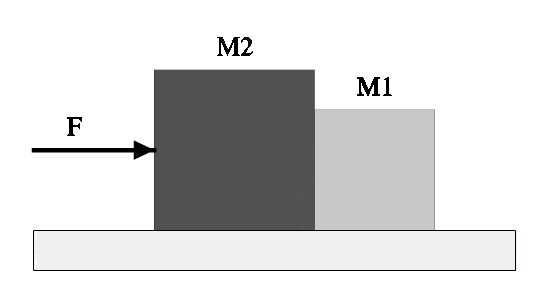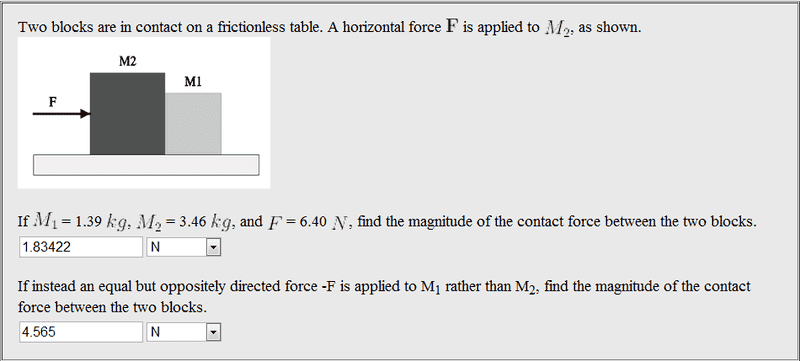# How do we get the formula for the contact force ?

My teacher said there isn't a special kind of formula, you just derive it from f=ma.

This is the diagram:Doc Al
Mentor
I assume you want the contact force between those two masses. Your teacher is right. Just apply Newton's 2nd law.

Hint: First find the acceleration of the system.

I assume you want the contact force between those two masses. Your teacher is right. Just apply Newton's 2nd law.

Hint: First find the acceleration of the system.

Yes. I found the acceleration.

Doc Al
Mentor
Yes. I found the acceleration.
Good. Now apply Newton's 2nd law to M1 alone.

To find the the answer in N ?

So-> F= m1 * acceleration that I found for the whole system ?

Ok, I found the answer. But scientifically, I don't understand how.
My teacher said that the contact force for both blocks is the same. How is that possible ? So if a bee flying at 10 km/h hits a car moving at 30 km/h the forces between both bodies is the same. How is that possible ?

Doc Al
Mentor
To find the the answer in N ?

So-> F= m1 * acceleration that I found for the whole system ?
Right.
Ok, I found the answer. But scientifically, I don't understand how.
My teacher said that the contact force for both blocks is the same. How is that possible ?
That's Newton's 3rd law. Whenever two objects interact, they exert equal and opposite forces on each other.
So if a bee flying at 10 km/h hits a car moving at 30 km/h the forces between both bodies is the same.
Definitely.

Man I love physics. lol.

Ok but why did we use m1 rather then m2 when we applied newtons second law on m1 ?

Doc Al
Mentor
Ok but why did we use m1 rather then m2 when we applied newtons second law on m1 ?
If you're applying Newton's 2nd law to m1, why in the world would you use m2?

SammyS
Staff Emeritus
Homework Helper
Gold Member
Draw two Free Body Diagrams, one for each block

Yes but here is what we did:

We found acceleration for the whole system.

Find the magnitude of the contact force between the two blocks.

Why would you use the mass of m1 and not m2 ?

Doc Al
Mentor
Why would you use the mass of m1 and not m2 ?
When applying Newton's 2nd law to m1, use the mass of m1.

You can also apply Newton's 2nd law to m2, in which case you'll use the mass of m2.

You'll get the same answer for the contact force either way. (Analyzing m1 is a bit easier, since only one force acts.)

Here's the problem to make it clearer:My acceleration is: 1.32 m/s^2

And then I just multiplied 1.32 m/s^2 by 1.39 m/s^2.
If I do 1.32 m/s^2 *3.46 m/s^2 I don't get the same answer...

Doc Al
Mentor
My acceleration is: 1.32 m/s^2
Right.
And then I just multiplied 1.32 m/s^2 by 1.39 m/s^2.
That's correct.
If I do 1.32 m/s^2 *3.46 m/s^2 I don't get the same answer...
The contact force is not the only force acting on m2. To apply Newton's 2nd law to m2, you must use the net force: ƩF = m2*a.

I get it. Thanks.

Can you help me to draw a FBD for this problem ?

Doc Al
Mentor
Can you help me to draw a FBD for this problem ?
Sure. First identify the forces acting on each block. What forces act on m2? On m1?

Sure. First identify the forces acting on each block. What forces act on m2? On m1?

Not sure but

m1-> force coming from m2 and force going forward.

m2->force from F (6.40N) and force of contact.

Doc Al
Mentor
m1-> force coming from m2 and force going forward.
What do you mean by 'force going forward'? The only thing in contact with m1 is m2.
m2->force from F (6.40N) and force of contact.
Right. They act in different directions, of course.

<-m1

<-m2->

or

<-m1->

<-m2->

Doc Al
Mentor
<-m1

<-m2->

or

<-m1->

<-m2->
Only one force acts on m1. In what direction does it act?

Well there is the force of contact... Does this count ?

I GOT IT !!

Thanks !

but just a last question-> for m2 is it 6.20 + F = 2.82 *1.18 OR -6.20 + F = 2.82 *1.18

Doc Al
Mentor
but just a last question-> for m2 is it 6.20 + F = 2.82 *1.18 OR -6.20 + F = 2.82 *1.18
I don't quite understand what you are doing here. You want:

ƩF = m2*a

The two forces are the applied force "F" which acts to the right and the contact force "Fc" which acts to the left. If you choose a sign convention so that the right is positive, then you have:

+F -Fc = m2*a

6.2 - Fc = 3.46*1.32

And so on.

How would the FBD look like for m2 ?

Doc Al
Mentor
How would the FBD look like for m2 ?
The applied force F acting to the right; the contact force from m1 acting to the left.

But where are the arrow pointing ?

<-m2->

Like this ?

Doc Al
Mentor
But where are the arrow pointing ?

<-m2->

Like this ?
That doesn't specify which arrow goes with which force. I'd draw it like this:
Code:
<---(Fc)---[m2]---(F)--->

ok sounds good. Thanks for your help. Very good teaching skills.